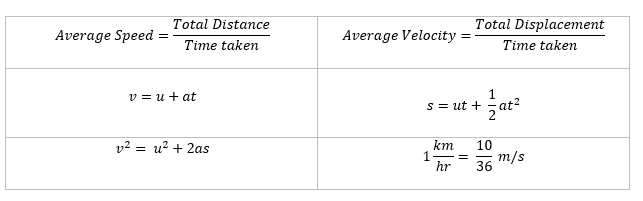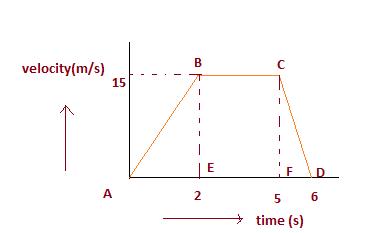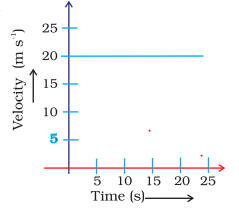In this page we have Numerical Questions and answers on Motion for Class 9 physics .Hope you like them and do not forget to like , social share and comment at the end of the page.
Formula usedQuestion 1
A train accelerates from 36 km/h to 54 km/h in 10 sec.
(i) Acceleration
(ii) The distance travelled by car.

a. Acceleration is given by
$a = \frac{{\Delta v}}{{\Delta t}}$
$\Delta v = 54 -36 = 18 km/hr =\frac {18 \times 1000}{3600} m/s= 5 m/s$
So a=.5 m/s2
b. Distance is given by
$S = ut + \frac{1}{2}a{t^2}$
Now u=36 km/hr =10m/s
$s=10 \times 10 + \frac {1}{2} \times .5 \times 10^2$
So s=125m

Question 2
A body whose speed is constant
(a) Must be accelerated
(b) Might be accelerated
(c) Has a constant velocity
(d) Cannot be accelerated.

might be accelerated

Question 3
A truck travelling at 54 km/h is slow down to 36 km/h in 10 sec. Find the retardation

Here u=54 km/h =15 m/s ,v=36 km/h=10 m/s
Acceleration is given by
$a = \frac{{\Delta v}}{{\Delta t}}$
$a = \frac {10 -15}{10} =- .5$
So a=-.5 m/s2
Negative sign implies retardation

Question 4
A particle is moving in a circle of diameter 20 m. What is its distance and as per the table given below
S.no Rounds Displacement Distance
1 1
2 1.5
3 2
4 2.5

After every round, particle comes to it starting position. So displacement at full rounds will be zero

S.no Rounds Displacement Distance
1 1 0 $20\pi$
2 1.5 20 m $30\pi$
3 2 0 $40\pi$
4 2.5 20m $50\pi$

Question 5
A scooter travelling at 10 m/s speed up to 20 m/s in 4 sec. Find the acceleration of scooter.

Acceleration is given by
$a = \frac{{\Delta v}}{{\Delta t}}$
$a = \frac {20 -10}{4} = \frac {10}{4}=2.5$
So a=2.5 m/s2

Question 6
A train starts from rest and accelerate uniformly at the rate of 5 m/s2 for 5 sec. Calculate the velocity of train in 5 sec.

Here u=0 ,a= 5m/s2 ,t= 5 sec,v=?
Now $v=u+at$
$v= 0 + 5 \times 5 =25$ m/s

Question 7
A object moves with uniform positive acceleration. Its velocity-time graph will be
(a) A straight line parallel to the time axis
(b) A straight line inclined at an obtuse angle to the time axis
(c) A straight line inclined at an acute angle to the time axis
(d) None of these.

Question 8
The maximum speed of a train is 90 km/h. It takes 10 hours to cover a distance of 500 km. Find the ratio of its average speed to maximum speed?

$\text {Average speed}= \frac {\text {total distance}}{time} =\frac {500}{10}=50$ km/hr
Ratio of average speed to maximum speed= 50:90=5:9

Question 9
A car start from rest and acquire a velocity of 54 km/h in 2 sec. Find
(i) the acceleration
(ii) distance travelled by car assume motion of car is uniform?

u=0, v=54 km/h=15 m/s ,t=2 sec
a) Acceleration is given by
$a = \frac{{\Delta v}}{{\Delta t}}$
$a = \frac {15-0}{2}$ So a=7.5 m/s2
b) Distance is given by
$S = ut + \frac{1}{2}a{t^2}$
$s= 0 \times 2 + \frac{1}{2} \times 7.5 \times 4$
s=15m

Question 10
An object dropped from a cliff falls with a constant acceleration of 10 m/s2. Find its speed 5 s after it was dropped.

$v=u+at$
$u = 0$
$v = 10 \times 5 = 50m/s$

Question 11
A ball is thrown upwards and it goes to the height 100 m and comes down
1) What is the net displacement?
2) What is the net distance?

As it comes down to the initial point
Net displacement is zero
Net distance=200 m

## Practice questions on motion

Question 12
Two cars A and B race each other. The Car A ran for 2 minute at a speed of 7.5 km/h, slept for 56 minute and again ran for 2 minute at a speed of 7.5 km/h. find the average speed of the car A in the race.

We know that
$Distance = speed \times time$
Distance travelled in first 2 minute = $\frac {7.5 \times 2}{60}$ = 0.25 km

Distance travelled in last 2 minute = $\frac {7.5 \times 2}{60}$= 0.25 km

Total distance = .25+.25 = 0.5 km
Total time = 2+2+56 = 60 minute = 1 hr

Average speed = 0.5/1
= 0.5 km/hr

Question 13
Anand leaves his house at 8.30 A.M for his school. The school is 2 km away and classes start at 9.00 A.M. If he walks at a speed of 3 km/h for the first kilometre, at what speed should he walk the second kilometre to reach just in time?

$speed =\frac {distance}{time}$
or
$time= \frac {distance}{speed}$
For travelling 1 km with 3 km/hr,
Time taken = 1/3 hr = 20 min
Now he has to reach school in 30 min,So he has cover another 1 km in = 30 -20 =10 min= 1/6 hr
So speed should = distance /time= 1/(1/6) = 6 km/hr

Question 14
An object moves along a straight line with an acceleration of 2 m/s2. If its initial speed is 10 m/s, what will be its speed 2 s later?

u=10 m/s,t=2 s, a=2 m/s2
$v=u+at$
$v= 10 + 2 \times 2 =14$ m/s

Question 15
A bullet hits a Sand box with a velocity of 20 m/s and penetrates it up to a distance of 6 cm. Find the deceleration of the bullet in the sand box.

We are given the following information:
Initial velocity, $u = 20 \, \text{m/s}$
Distance traveled, $d = 6 \, \text{cm} = 0.06 \, \text{m}$
We can use the equation of motion: $v^2 = u^2 + 2ad$ Since the bullet comes to rest ($v = 0 \, \text{m/s}$), we have: $0 = u^2 + 2ad$ Solving for deceleration ($a$): $2ad = -u^2$ $a = \frac{{-u^2}}{{2d}}$ Substituting the given values: $a = \frac{{-(20 \, \text{m/s})^2}}{{2 \cdot 0.06 \, \text{m}}}$ $a = -\frac{{400 \, \text{m}^2/\text{s}^2}}{{0.12 \, \text{m}}}$ $a = -3333.33 \, \text{m/s}^2 \, \text{(rounded to two decimal places)}$ Therefore, the deceleration of the bullet in the sand box is approximately $-3333.33 \, \text{m/s}^2$.

Question 16
A particle experiences constant acceleration for 20 seconds after starting from rest. If it travels a distance D1 in the first 10 seconds and distance D2 in the next 10 seconds then,
(a) D2 = D1
(b) D2 = 2D1
(c) D2 = 3D1
(d) D2 = 4D1

(c)

Question 17
The velocity -time graph of SUV is given below. The mass of the SUV is 1000 kg.a. What is the distance travelled by the SUV is first 2 seconds?
b. What is the braking force at the end of 5 seconds to bring the SUV to a stop within one seconds?

a. The distance travelled by the SUV is first 2 seconds = Area of $\Delta ABE$
$= \frac {1}{2} \times AE \times BE$
$=\frac {1}{2} \times 2 \times 15= 15m$
b. Acceleration will be given by the slope of the line CD
$a= \frac {0 -15}{6-5} = -15 m/s^2$
Now mass of the SUV =1000 Kg
Braking Force will be
$F=ma = 1000 \times -15 =-15000 N$

Question 18
An electron moving with a velocity of $5 \times 10^3 m/s$ enters into a uniform electric field and acquires a uniform acceleration of $10^3 m/s^2$ in the direction of initial velocity.
i. Find out the time in which electron velocity will be doubled.?
ii. How much distance electron would cover in this time.?

Given $u= 5 \times 10^3 m/s$ , $a=10^3 m/s^2$, $v=2u =10^4$ m/s
i.Using $v=u+at$
$10^4 = 5 \times 10^3 + 10^3 t$
or
t=5 sec
ii. Using $s=ut+ \frac {1}{2}at^2$
$s = 5 \times 10^3 \times 5 + \frac {1}{2} \times 10^3 \times 5^2$
$=37.5 \times 10^3 m$

Question 19
A train 100 m long is moving with the velocity of 72 km/hr. Find the time it takes to cross the bridge which is 2 km long?

Given Lenght of Train = 100m , velocity = 72 km/hr = 20 m/s, Lenght of the Bridge =2 Km.
Total distance covered by the train to fully pass the bridge = 2000 + 100 = 2100 m
So, time taken
$time = \frac {distance}{velocity} = \frac {2100}{20} =105 sec$

Question 20
An artificial satellite is moving in a circular orbit of radius 42.250 km (approx). Calculate its linear velocity if takes 24 hour to revolve around earth.?

Given r =42.250 km , T= 24 hour
Linear velocity in circular motion is given by
$v = \frac {2 \pi r}{T} = \frac {2 \times 3.14 \times 42.250}{24} = 11.05 km/hr$

Question 21
The velocity-time graph shows the motion of a cyclist. Find
(i) its acceleration
(ii) its velocity
(iii) the distance covered by the cyclist in 15 seconds(i) We can see from the graph that velocity is not changing So,acceleration is equal to zero.
(ii) By Reading the graph, velocity = 20 m/s
(iii) Distance covered in 15 seconds, $s = u \times t = 20 \times 15 = 300$ m

Question 22
An object starting from rest travels 20 m in first 2 s and 160 m in next 4 s.What will be the velocity after 7 s from the start.

Here u=0, s=20 m ,t=2 sec
$S = ut + \frac{1}{2}a{t^2}$
$20= 0 + \frac {1}{2} a \times 4$
$a=10 m/s^2$
Now Velocity at the end of 2 sec
$v=u+at$
$v=10 \times 2=20$ m/s
Now Checking the motion for next 4 sec, let assume acceleration be $a_1$ as we are not sure about the motion in second part
Here u=20 m/s ,s=160 m ,t=4 sec
$S = ut + \frac{1}{2}a{t^2}$
$160 = 20 \times 4 + \frac {1}{2} a_1 \times 16$
$a_1 = 10 m/s^2$
So acceleration is constant in both the motion.
Now we can easily calculate velocity as
u=0, t= 7sec , a=10m/s2
$v=u+at$
$v= 0 + 10 \times 7= 70 m/s$

Question 23
An object travels 20 m in 2 s and then another 16 m in 2 s. What is the average speed of the object?

Total distance travelled by the object =20 m + 16 m = 36 m
Total time taken = 2 s + 2 s = 4 s
$\text {Average Speed} = \frac {\text {total Distance travelled}}{\text {total time taken }}$
$\text {Average Speed} = \frac {36}{4} = 9$ m/s

Question 24
The distance moved and the magnitude of displacement are equal in ?
(a) The earth is revolving around the Sun
(b) Object moving in circular path
(c) The pendulum is moving to and fro
(d) Object moving on straight road

(d) only.
Distance and displacement in all other cases

Question 25
Stopping distance of vehicles : When brakes are applied to a moving vehicle, the distance it travels before stopping is called stopping distance. It is an important factor for road safety and depends on the initial velocity ($v_0$) and the braking capacity, or deceleration, ?a that is caused by the braking. A car travelling at speed 72km/hr suddenly applies the brake with the deceleration of 5m/s2. Find the stopping distance of the car

Here u=72 km/hr = $\frac {72 \times 1000}{3600} m/s= 20$ m/s , v=0
a= -5 m/s2
Now using the relation
$v^2 = u^2 + 2as$
$0 = (20)^2 + 2 \times (-5) \times s$
$s= 40 m$

## Summary

This numericals of motion class 9 with answers is prepared keeping in mind the latest syllabus of CBSE . This has been designed in a way to improve the academic performance of the students. If you find mistakes , please do provide the feedback on the mail. You can download this as PDF also